# ISEE Upper Level Math : How to find the length of the side of an equilateral triangle

## Example Questions

### Example Question #1 : Equilateral Triangles

For an equilateral triangle, Side A measures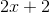and Side B measures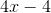. What is the length of Side A?

4

3

10

8

8

Explanation:

First you need to recognize that for an equilateral triangle, all 3 sides have equal lengths.

This means you can set the two values for Side A and Side B equal to one another, since they measure the same length, to solve for.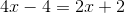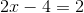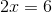You now know that, but this is not your answer. The question asked for the length of Side A, so you need to plug 3 into that equation.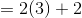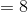So the length of Side A (and Side B for that matter) is 8.

### Example Question #2 : Equilateral Triangles

One angle of an equilateral triangle is 60 degrees. One side of that triangle is 12 centimeters. What are the measures of the two other angles and two other sides?

45 degrees, 75 degrees, 12 centimeters, 13 centimeters

90 degrees, 30 degrees, 12 centimeters, 12 centimeters

55 degrees, 65 degrees, 12 centimeters, 13 centimeters

60 degrees, 60 degrees, 12 centimeters, 12 centimeters

50 degrees, 70 degrees, 5 centimeters, 12 centimeters

60 degrees, 60 degrees, 12 centimeters, 12 centimeters

Explanation:

An equilateral triangle is one in which all three sides are congruent. It also has the property that all three interior angles are equal. In other words, all three angles of an equilateral triangle are always 60°.  Since all sides are congruent, the other two sides both measure 12 centimeters.

### Example Question #3 : Equilateral Triangles

If an equilateral triangle has a perimeter of 18in, what is the length of one side?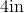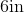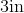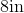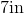Explanation:

To find the perimeter of an equilateral triangle, we will use the following formula: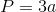where a is the length of any side of the triangle.  Because an equilateral triangle has 3 equal sides, we can use any side in the formula.  To find the length of one side of the triangle, we will solve for a.

Now, we know the perimeter of the equilateral triangle is 18in.  So, we will substitute.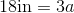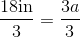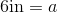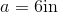Therefore, the length of one side of the equilateral triangle is 6in.

### Example Question #4 : Equilateral Triangles

An equilateral triangle has a perimeter of 39in.  Find the length of one side.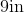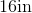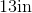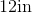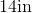Explanation:

An equilateral triangle has 3 equal sides.  So, to find the length of one side, we will use what we know.  We know the perimeter of the equilateral triangle is 39in.  So, we will look at the formula for perimeter.  We getwhere a is the length of one side of the triangle.  So, to find the length of one side, we will solve for a.  Now, as stated before, we know the perimeter of the triangle is 39in.  So, we will substitute and solve for a.  We get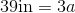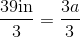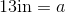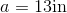Therefore, the length of one side of the equilateral triangle is 13in.